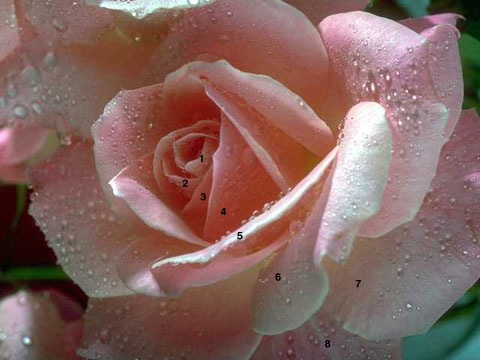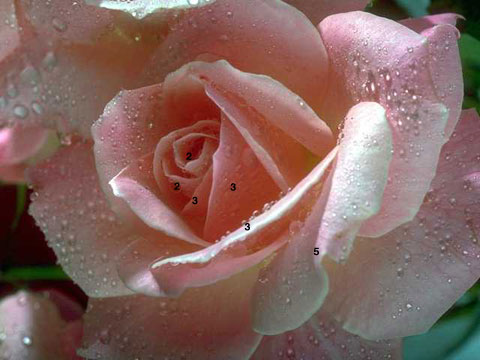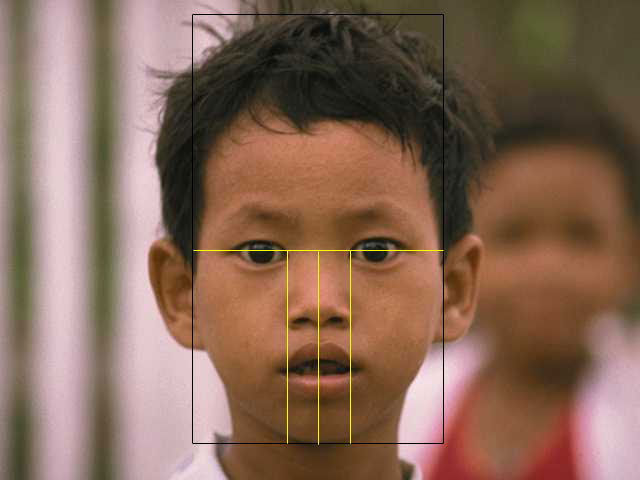SEARCH HOMEMath Central Quandaries & Queriespamela, a student: how do artists use integers?Hi Pamela,

The integers include 0, the whole numbers (1,2,3,4,5...) and their negatives (-1, -2, -3, -5, ...). Negative numbers and 0 are not used by artists from what I have seen but there is a special sequence of whole numbers called Fibonacci's Numbers that they do use. The sequence is 1, 1, 2, 3, 5, 8, 13, 21, ... where each term is found by adding the previous two terms. Artists use the Fibonacci numbers without realizing it because these are numbers that found so often in nature. For example, the number of rows of petals on a rose is a Fibonacci number, in this case 8 rows of petals:For the same rose, notice that the number of petals in each row are Fibonacci numbers:Artists also use a ratio derived from the Fibonacci numbers called the golden ratio which is approximately 1.6180339887. They use the ratio without realizing it because it is pleasing to the eye. The face is a perfect example of the Golden Ratio or Divine Proportion. The head forms a golden rectangle with the eyes at its midpoint. The mouth and nose are each placed at golden sections of the distance between the eyes and the bottom of the chin. A golden rectangle is a rectangle whose side lengths are in the golden ratio, 1:φ (one-to-phi), that is, approximately 1:1.618.There is so much more on this topic. I suggest an Internet search on "Divine Proportion", "Golden Ratio", "Rule of Thirds", "Golden Ratio" and "Fibonacci Numbers".

Hope this helps,

JaniceMath Central is supported by the University of Regina and The Pacific Institute for the Mathematical Sciences.